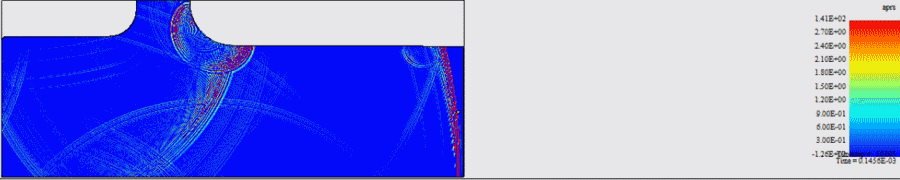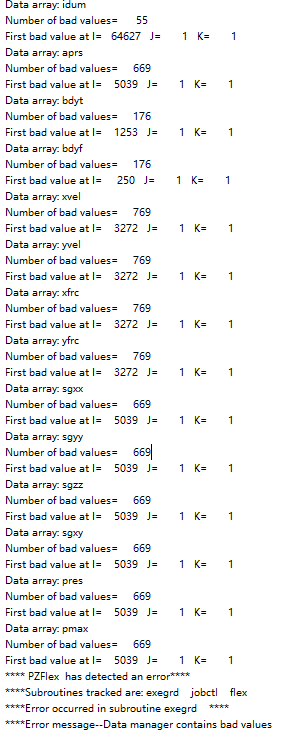# abnormal wave propagation and bad value error

Hi，

As the gif showing，the ultrasonic wave propagates to the boundary of the model and is absorbed，but a few seconds later，a wave of chaos  appears from the boundary. After the chaos wave propagated for a few seconds,the job finished abnormally and reported error of bad values.The following code is the list of nodes and the BC I set .

`          x1                   (  real   ) =    0.000000              x2                   (  real   ) =   0.8000000E-03          x3                   (  real   ) =   0.8608000              x4                   (  real   ) =   0.8618000              idx                  ( integer ) =          4          xmax                 (  real   ) =   0.8618000              y1                   (  real   ) =    0.000000              y2                   (  real   ) =   0.1625000E-01          y3                   (  real   ) =   0.3625000E-01          y4                   (  real   ) =   0.4625000E-01          y5                   (  real   ) =   0.6625000E-01          y6                   (  real   ) =   0.7625000E-01          y7                   (  real   ) =   0.9625000E-01          y8                   (  real   ) =   0.1062500              y9                   (  real   ) =   0.1262500              y10                  (  real   ) =   0.1362500              y11                  (  real   ) =   0.1562500              y12                  (  real   ) =   0.1662500              y13                  (  real   ) =   0.1862500              y14                  (  real   ) =   0.1962500              y15                  (  real   ) =   0.2162500              y16                  (  real   ) =   0.2262500              y17                  (  real   ) =   0.2462500              jaend                ( integer ) =         17          y18                  (  real   ) =   0.3300000              jdx                  ( integer ) =         18          ymax                 (  real   ) =   0.3300000              i1                   ( integer ) =          1          i2                   ( integer ) =          9          i3                   ( integer ) =       8072          i4                   ( integer ) =       8081          indgrd               ( integer ) =       8081          j1                   ( integer ) =          1          j2                   ( integer ) =        153          j3                   ( integer ) =        341          j4                   ( integer ) =        435          j5                   ( integer ) =        622          j6                   ( integer ) =        716          j7                   ( integer ) =        903          j8                   ( integer ) =        997          j9                   ( integer ) =       1184          j10                  ( integer ) =       1278          j11                  ( integer ) =       1465          j12                  ( integer ) =       1559          j13                  ( integer ) =       1746          j14                  ( integer ) =       1840          j15                  ( integer ) =       2027          j16                  ( integer ) =       2121          j17                  ( integer ) =       2308          j18                  ( integer ) =       3093          jndgrd               ( integer ) =       3093`
`boun	side xmin free	defn x860 absr	node \$i3 \$i3 \$j1 \$j18	defn x861 absr	node \$i3+1 \$i3+1 \$j1 \$j18	side xmax absr	side ymin free	side ymax absrend	`

Could please explain the reason and how to set the BC? If need,I can share my input file.

Best  regards

By the way , I posted two yesterday, but still pending approval.Please pass my posts and look forward to  the answer and reply.

### 3件のコメント

• 正式なコメント

Hi,

Try switching out to IMPD.

Thanks,

Kevin

• Can you share the model files with us? Maybe an image of the geometry and where you would like to place the absorbers would help.

Oliver

• Hi,